# Paths

Relevant articles: .

# Paths

The path with$m$ vertices is denoted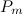$P_m$. Note that paths are trees.

## Spectrum Results

The spectrum problem for paths was completely settled by Tarsi in .

Theorem 1 

Let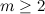$m\geq 2$. There exists a$P_m$-design of order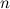$n$ if and only if

•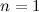$n=1$ or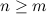$n \geq m$; and
•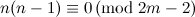$n(n-1)\equiv 0 \,({\rm mod \ }2m-2)$.

## References

1. 1.0 1.1 1.2 Tarsi, M. Decomposition of a complete multigraph into simple paths: nonbalanced handcuffed designs, J. Combin. Theory Ser. A, 34, 60–70 (1983).